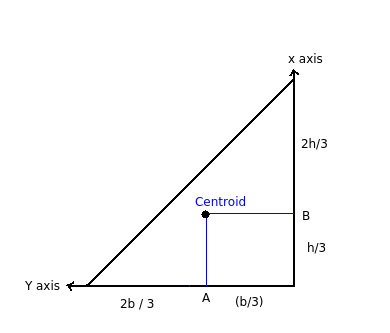# Right angled triangle - Laminas not having centroid at its geometrical centre

Q.  Which of the following laminas do not have centroid at its geometrical centre?
- Published on 18 Sep 15

a. Circle
b. Equilateral triangle
c. Right angled triangle
d. None of the above

#### Discussion

• Ishant   -Posted on 26 Feb 20
I am commenting here for a little more clarification from your side because I got this question on my U.G assignment.
Thanks
• Ishant   -Posted on 26 Feb 20
In your second point you mentioned that centroid is definedas the geometrical centre of body. This clearly explains why every 2-d object should have same point as it's centroid as it's geometrical centre.
It can be conversely said that geometrical centre of a body can be defined as the centroid of the body but centroid for 2-d bodies is also defined as the point about which the first moment of area is zero.
• Emani aditya santhosh   -Posted on 18 Aug 19
Hi
• Sravanthi   -Posted on 14 Dec 15
- Right angled triangles do not have centroid at its geometrical centre.

- Centroid is defined as the geometrical centre of a body. The position of center of mass and centroid are identical if the density of material is uniform throughout the body.

- Centroid is the point of intersection of three medians in a triangle. Median is a line segment joining midpoint of a side and opposite vertex.- Masses in non-uniform body are randomly distributed. Hence, in these objects it is observed at the centre of gravity shifts from the centroid point. But for the body having uniform density, centre of gravity lies on the geometrical centre.

- Circle, square and equilateral triangles have geometrical center as centroid, but this is not possible for a right angled triangle.

## ➨ Post your comment / Share knowledgeEnter the code shown above:

(Note: If you cannot read the numbers in the above image, reload the page to generate a new one.)